Courses

# Regular Expressions And Languages Practice Quiz - 1

## 20 Questions MCQ Test RRB JE for Computer Science Engineering | Regular Expressions And Languages Practice Quiz - 1

Description
This mock test of Regular Expressions And Languages Practice Quiz - 1 for Computer Science Engineering (CSE) helps you for every Computer Science Engineering (CSE) entrance exam. This contains 20 Multiple Choice Questions for Computer Science Engineering (CSE) Regular Expressions And Languages Practice Quiz - 1 (mcq) to study with solutions a complete question bank. The solved questions answers in this Regular Expressions And Languages Practice Quiz - 1 quiz give you a good mix of easy questions and tough questions. Computer Science Engineering (CSE) students definitely take this Regular Expressions And Languages Practice Quiz - 1 exercise for a better result in the exam. You can find other Regular Expressions And Languages Practice Quiz - 1 extra questions, long questions & short questions for Computer Science Engineering (CSE) on EduRev as well by searching above.
QUESTION: 1

### Which one of the following regular expressions represents the language: the set of all binary strings having two consecutive 0's and two consecutive 1's?

Solution:

Set of all binary strings having two consecutive 0s and two consecutive1s

Anything 00 Anything 11 Anything + Anything 11 Anything 00 Anything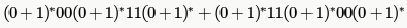And it is same after taking common.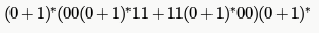Neither they said Both are immediate nor they give a predefined order, so it should be as above

*Multiple options can be correct
QUESTION: 2

### Choose the correct alternatives (more than one may be correct) and write the corresponding letters only. Let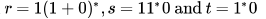be three regular expressions. Which one of the following is true?

Solution:

to know the ans let us check all the options.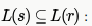strings generated by S are any numbers of 1's followed by one 0, i.e., 10,110,1110,1110,......) Strings generated by r are is 1 followed by any combination of 0 or 1, i.e., 1,10,11,1110,101,110,.....This shows that all the strings that can be generated by S,can also be generated by r it means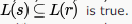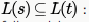here strings generated by t are any numbers of 1 (here 1* means we have strings as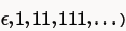followed by only one 0, i.e., 0,10,110,1110,.....So we can see that all the strings that are present in S can also be
generated by t, hence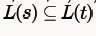which shows that option A is true.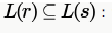this is false because string 1 which can be generated by r, cannot be generated by S

Same as option A.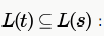. this is false because string 0 which can be generated by t ,cannot be generated by S .

QUESTION: 3

### Choose the correct alternatives (more than one may be correct) and write the corresponding letters only: Which of the following regular expression identities is/are TRUE?

Solution:

(b) RHS generates ∑* while LHS can't generate strings where r comes after s like sr,srr etc. LHS ⊂ RHS.

(c) LHS generates ∑* while RHS can't generate strings where r comes after an S. RHS  ⊂ LHS.

(d) LHS contains all strings where after an s, no r comes. RHS contains all strings of eitherr or s but no combination of them. So, RHS ⊂ LHS.

QUESTION: 4

In some programming language, an identifier is permitted to be a letter followed by any number of letters or digits. If  and  denote the sets of letters and digits respectively, which of the following expressions defines an identifier?

Solution:

It has to be started by a letter followed by any number of letters and digits.

QUESTION: 5

Which two of the following four regular expressions are equivalent? (ε is the empty string).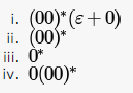Solution:

you can have any no. of 0's as well as null.
A is false because you cannot have single 0 in ii). same for option B. In D you are forced to have single 0 in iv) whereas not in iii).

QUESTION: 6

Which one of the following regular expressions over {0,1} denotes the set of all strings not containing  as substring?

Solution:

"A regular expression denoting a language (set of strings) means it should generate all string in L and notgenerate any string not in L"

(a) - generates 100
(b) doesn't generate 0 (start trying strings in lexicographic order- 0, 1, 00, 01, 10,...)
(c) doesn't generate 1

QUESTION: 7

If the regular set A is represented by A — (01 + 1)* and the regular set B is represented by B — ((01)*1*)*, which of the following is true?

Solution:

Both generates all strings over {0,1} where a 0 is immediately followed by a 1.

*Multiple options can be correct
QUESTION: 8

The string 1101 does not belong to the set represented by

Solution:

Only (a) and (b) can generate 1101.

R.E of option C won’t generate 1101 as you can see the language will contain L(C) = {epsilon,10,1010,1001,0101,00,11,0011,1100,………..}
Also, R.E of option D has ‘1’ but here two ’11’ are together hence its impossible to generate 1101.L(D) = {Epsilon,0,00,110,11110,11000,…………….}

QUESTION: 9

Lets' and T be languages over S = {a. b} represented by the regular expressions (a + b*)* and (a + b)*, respectively. Which of the following is true?

Solution:

Both generates all strings over ∑ .

QUESTION: 10

The regular expression 0*(10*)* denotes the same set as

Solution:

(A) is the answer. Both (A) and the given expression generates all strings over ∑ .

(B) doesn't generate 11

(C) doesn't generate 11

QUESTION: 11

Which of the following statements is TRUE about the regular expression 01*0?

Solution:

Infinite set (because of *) of finite strings. A string is defined as a FINITE sequence of characters and hence can never be infinite.

QUESTION: 12

Which one of the following regular expressions is NOT equivalent to the regular expression (a + b + c)*?

Solution:

A) (a* + b* + c*)*  = ( ^ + a+aa+.. ..+b+bb+...+c+cc...)* = (a+b+c+ aa+..+bb+..+cc+..)*= (a+b+c)*  [any combination of rest of aa ,bb,cc, etc already come in (a+b+c)* ]

(a*b*c*)* = (a*+b*+c* +a*b*+b*c*+a*c*+..)*= (a+b+c+....)* = (a+b+c)*

((ab)* + c*)* =(ab+c+^+abab+...)* = (ab+c)*

(a*b* + c*)* = (a*+b*+c*+...)* =(a+b+c+..)* = (a+b+c)*

QUESTION: 13

Which regular expression best describes the language accepted by the non-deterministic automaton below?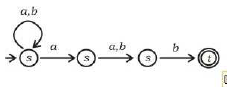Solution:

Say s1, s2 , s3 and t are states (in sequence)

s1 is start state
s1= ^ + s1a + s1b = ^+s1(a+b) = (a+b)* [using arden's theorem  R= Q+RP , then R= QP*][^ bcoz of start state]
s2 = s1a = (a+b)*a

s3= s2a+s2b = s2(a+b) = (a+b)*a(a+b)

t= s3b= (a+b)*a(a+b)b

t is final state so regular expression is (a+b)*a(a+b)b

QUESTION: 14

Consider the regular expression R = (a + b)* (aa + bb) (a + b)*

Which one of the regular expressions given below defines the same language as defined by the regular expression R?

Solution:

R = (a+b)*(aa+bb)(a+b)*

having NFA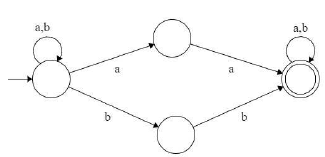Equivalent DFA :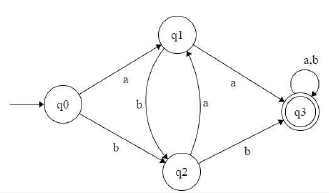which is equivalent Transition graph [ by removing transition from q1 to q2 and q2 to q1 but does not effect on language ..be careful ]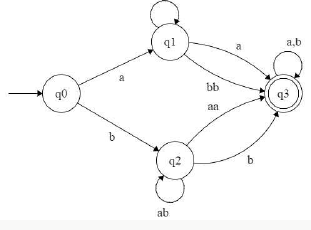That is equivalent to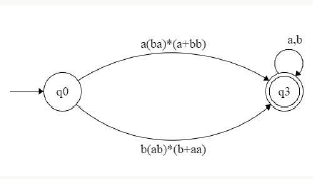which is equivalent to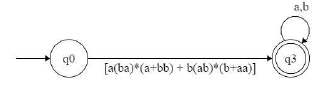which is equivalent to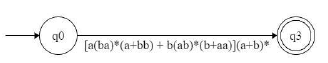so equivalent regular expression is [a(ba)*(a+bb) + b(ab)*(b+aa)](a+b)* so option C is answer.

QUESTION: 15

Which of the following regular expressions describes the language over{0, 1} consisting of strings that contain exactly two 1's?

Solution:

A) with at least 2 consecutive 1's, any no of 0's and any no of 1's

B) exactly two consecutive 1's

C)exactly two 1's but need not be consecutive

D) any no of 1's and 0's with at least two 1's

QUESTION: 16

Which one of the following languages over the alphabet {0,1} is described by the regular expression: (0 + 1)*0(0 + 1)*0(0 + 1)*?

Solution:

Counter example for other choices:

(A) 1010 is accepted which doesn't contain 00

(B) 000 is accepted

(D) 01 is not accepted

QUESTION: 17

Let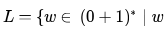has even number of 1s}  i.e., L is the set of all the bit strings with even numbers of 1s. Which one of the regular expressions below represents L?

Solution:

(A) - If the string contains a 1, it must end in a 1 hence cannot generate all bit strings with even number of 1's (eg, 1010)
(C) - between the second and third 1's a 0 is not allowed (eg, 011011)

(D) - 00 is not allowed, zero is an even number.

QUESTION: 18

Which of the regular expressions given below represent the following DFA?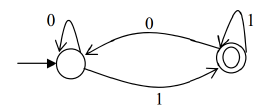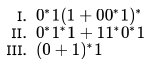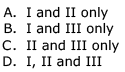Solution:

(B) is the answer. (II) doesn't generate 11011 which is accepted by the given DFA

QUESTION: 19

Let r,s,t be regular expressions. Which of the following identities is correct?

Solution:

a. (r + s)* = r*s*            LHS can generate 'sr' but RHS not

b. r(s + t) = rs + t          LHS can generate 'rt' but RHS not

c. (r + s)* = r* + s*       LHS can generate 'sr' but RHS not

d. (rs + r)* r = r (sr + r)*   They are equivalent

e. (r*s)* = (rs)*            LHS can generate 'rrrs' but RHS not

QUESTION: 20

Which of the following regular expressions correctly accepts the set of all 0/1-strings with an even (poossibly zero) numbe of 1s?

Solution:

As, mentioned in the question, the regular expression must accept all strings of 0 and 1 but with even no of 1s (including no 1s). Hence, 00 must be in the language. Option a, b, c, d do not accept 00. Hence, option e is correct.